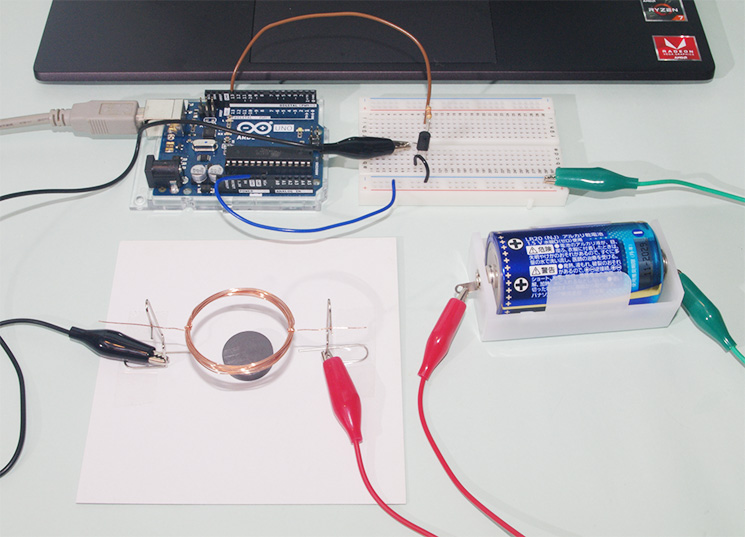## 用Raspberry Pi和SensorMedal制作IoT跳绳设备 第二部分：用SensorMedal检测跳跃次数并在显示器上显示## 本部分所需部件

### 跳绳### ROHM SensorMedal（SensorMedal-EVK-002）### Raspberry Pi zero（Raspberry Pi Zero W）### FOUR LETTER LED PHAT## 1. 用SensorMedal进行跳绳计数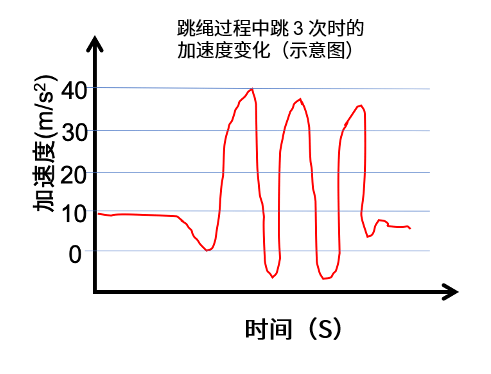```pi@raspizero:~ \$ sudo pip3 install bluepy
Looking in indexes: https://pypi.org/simple, https://www.piwheels.org/simple
Collecting bluepy
Installing collected packages: bluepy
Successfully installed bluepy-1.3.0

pi@raspizero:~ \$ sudo git clone http://github.com/bokunimowakaru/SensorMedal2
Cloning into 'SensorMedal2'...
Unpacking objects: 100% (78/78), done.

pi@raspizero:~ \$ cd SensorMedal2/
pi@raspizero:~/SensorMedal2 \$ sudo python3 ble_logger_SensorMedal2.py
Short Local Name = ROHMMedal2_0107_01.00
Flags = 06
Incomplete 16b Services = 0000180a-0000
Manufacturer = 0100c86abcdef
ID            = 0x1
SEQ           = 188
Temperature   = 27.99 ℃
Humidity      = 71.52 %
Pressure      = 998.578 hPa
Illuminance   = 93.3 lx
Accelerometer = 1.453 g ( 0.062 0.844 1.181 g)
Geomagnetic   = 92.6 uT ( -15.8 -58.0 -70.4 uT)
Magnetic      = 0x3
Steps         = 8 歩
Battery Level = 90 %
```

（每跳一次消耗的卡路里=270大卡/30*100次≒0.1大卡/次）

```pi@raspizero:~/SensorMedal2 \$ sudo cp ble_logger_SensorMedal2.py ../Programs/ble_jump.py

--- 下面的第5〜7、第12、第16〜18、第42〜55行是更改或添加部分 ---

interval = 1 #动作间隔
start_seq = 0
jump_cnt = 0

---
# 每个BLE设备的接收数据处理
for dev in devices:
isRohmMedal = False
sensors = dict()
for (adtype, desc, val) in dev.getScanData():
# print("  %s = %s" % (desc, val))
if desc == 'Short Local Name' and val[0:18] == 'ROHMMedal2_xxxx_xx': # 在xxxx_xx中输入您使用的SensorMedal编号
print(“JUMP!”)
isRohmMedal = True
if isRohmMedal and desc == 'Manufacturer':
---
# 在显示器上显示
print('    ID            =',sensors['ID'])
print('    SEQ           =',sensors['SEQ'])
“”” print('    Temperature   =',round(sensors['Temperature'],2),'℃')
print('    Humidity      =',round(sensors['Humidity'],2),'%')
print('    Pressure      =',round(sensors['Pressure'],3),'hPa')
print('    Illuminance   =',round(sensors['Illuminance'],1),'lx')
print('    Accelerometer =',round(sensors['Accelerometer'],3),'g (',\
round(sensors['Accelerometer X'],3),\
round(sensors['Accelerometer Y'],3),\
round(sensors['Accelerometer Z'],3),'g)')
print('    Geomagnetic   =',round(sensors['Geomagnetic'],1),'uT (',\
round(sensors['Geomagnetic X'],1),\
round(sensors['Geomagnetic Y'],1),\
round(sensors['Geomagnetic Z'],1),'uT)')
print('    Magnetic      =',sensors['Magnetic'])
print('    Steps         =',sensors['Steps'],'歩')
print('    Battery Level =',sensors['Battery Level'],'%')

SEQ = sensors['SEQ']
jump_cnt = sensors['Steps']

if SEQ in [0,1] and jump_cnt == 0:
start_seq+= 1
print(“START!")
else:
last_cnt = jump_cnt

if start_seq >= 1:
cur_cnt = jump_cnt
cur_cal = round(cur_cnt*0.1,1)
jump_text= str(cur_cnt)+" Jump "+str(cur_cal)+" Cal"
print(jump_text)

'''
for key, value in sorted(sensors.items(), key=lambda x:x):
print('    ',key,'=',value)
'''
```## 2. 在四位显示器上显示[ Pimoroni 四位显示器的 Github https://github.com/pimoroni/fourletter-phat ]```\$ curl https://get.pimoroni.com/fourletterphat | bash
```

```\$ python ~/Pimoroni/fourletter-phat/examples/clock.py
```## 3. 跳绳并显示次数

```pi@raspizero:~/Programs \$ sudo cp ble_jump.py ble_jump_4d.py

--- 下面的第4、11、12行为更改或添加部分 ---
import fourletterphat
---
if start_seq >= 1:
cur_cnt = jump_cnt
cur_cal = round(cur_cnt*0.1,1)
jump_text= str(cur_cnt)+" Jump "+str(cur_cal)+" Cal"
print(jump_text)
fourletterphat.print_number_str(cur_cnt)
fourletterphat.show()
'''
for key, value in sorted(sensors.items(), key=lambda x:x):
print('    ',key,'=',value)
'''
```## 4. 总结##### 吉田　显一（Yoshiken）

http://blog.ktrips.net

### 相关文章

1.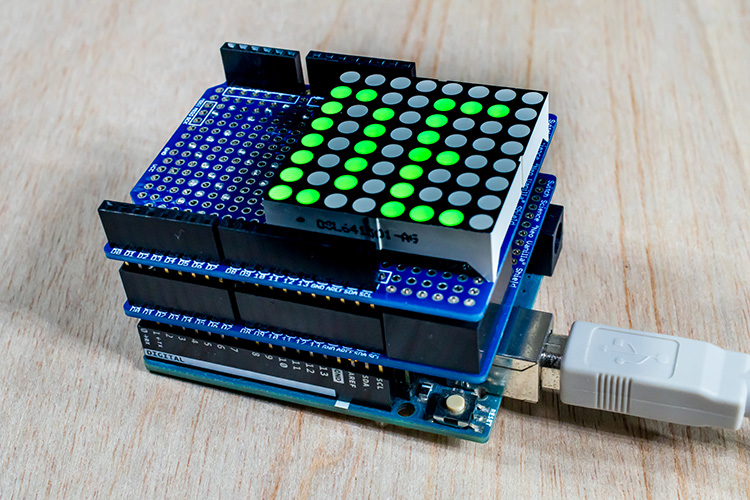#### 制作属于自己的Arduino扩展板！升级版Arduino电子制作

2.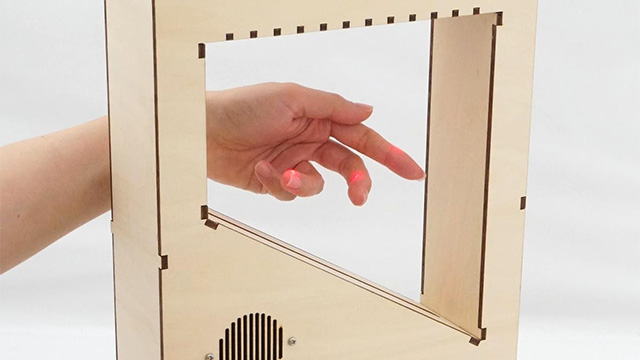#### 用Arduino和红光激光器制作激光琴【后篇】

3.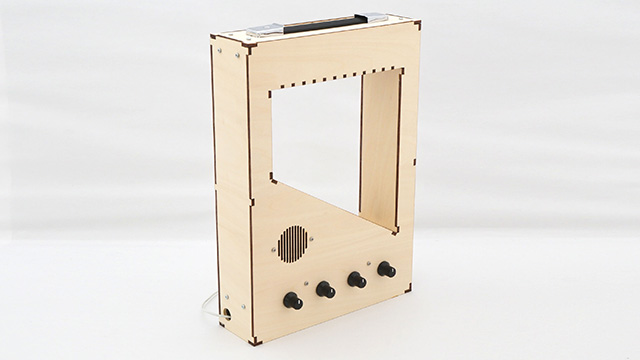#### 用Arduino和红光激光器制作激光琴【前篇】

4.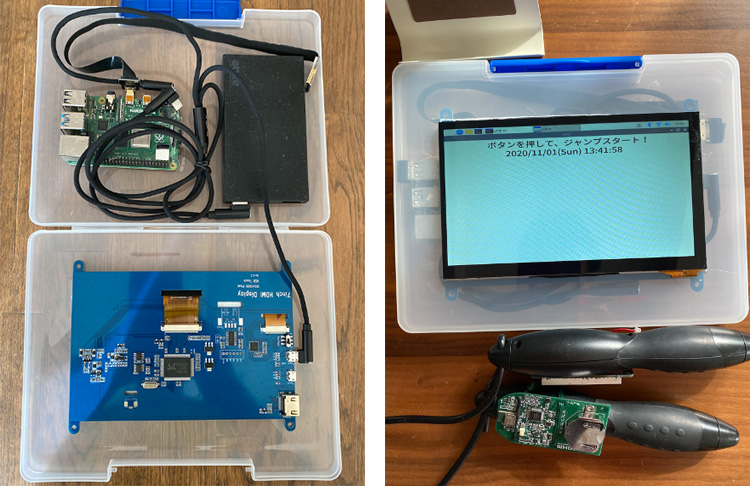#### 用Raspberry Pi和SensorMedal制作IoT跳绳设备　第四部分（剧终篇）：在设备上安…

5.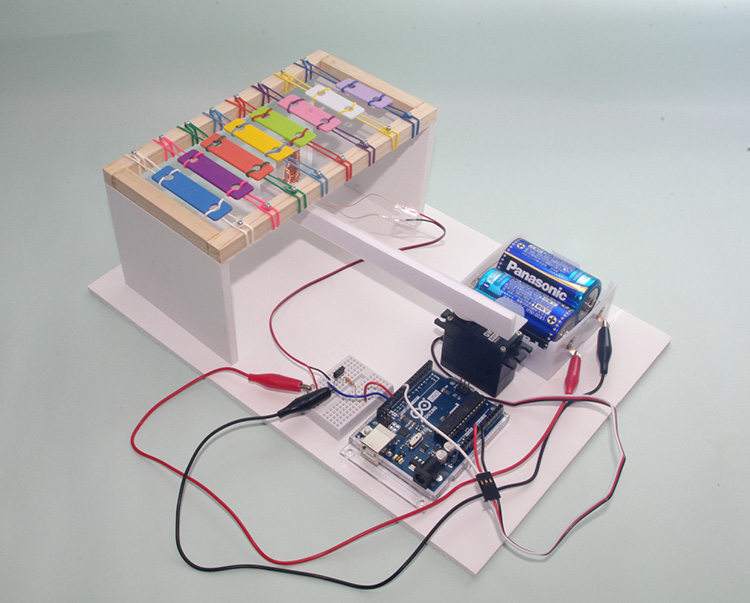#### 用Arduino作为控制设备让各种作品动起来吧！ 第5篇：用Arduino控制伺服电机来演奏乐器！

6.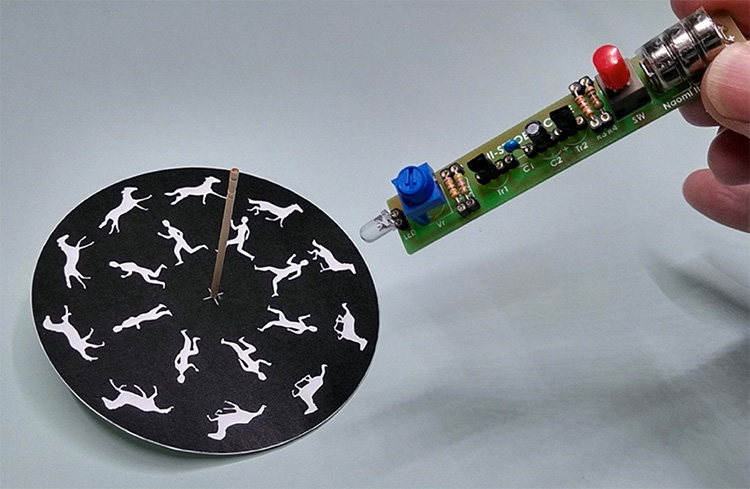#### 用Arduino作为控制设备让各种作品动起来吧！ 第4篇：享受控制RGB LED灯的乐趣

7.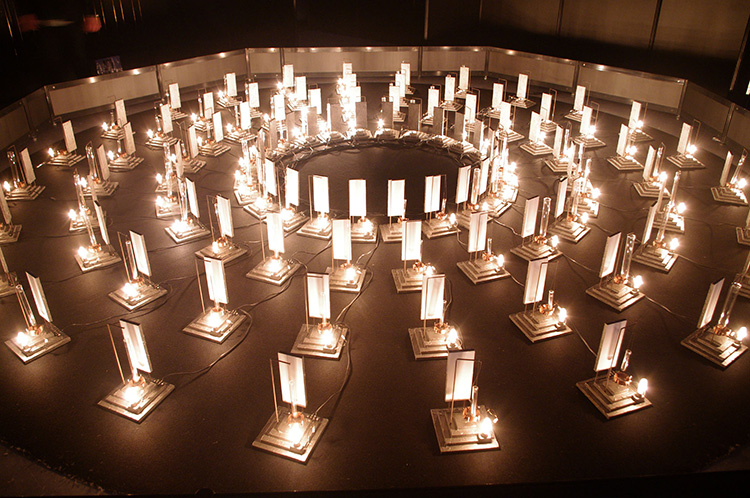#### 用Arduino作为控制设备让各种作品动起来吧！ 第3篇：电磁执行器的自制方法

8.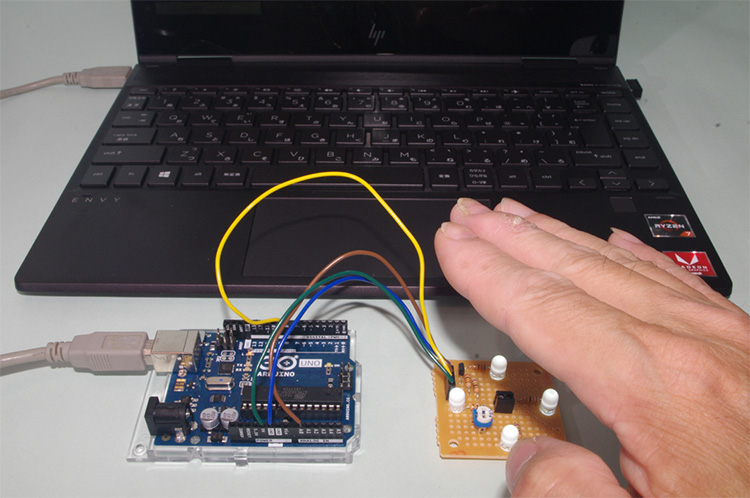#### 用Arduino作为控制设备让各种作品动起来吧！ 第2篇：非接触式开关的制作与控制

9.